Next: Diode Circuits Up: Filter Circuits Previous: Amplifier with Negative Feedback

# Problems

1. In the following circuit, the input signal is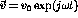, and the components have been chosen such that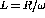and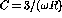. The output is at the terminals AB.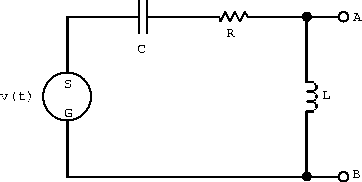1. What is the transfer function H()?
2. Find the current in the circuit.
3. What is the voltage drop across each element of the circuit?
4. Show algebraically that at any instant the potential difference around the circuit is zero.
5. At time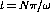, where N=0,2,4,... make a sketch showing the voltage across each element in the complex plane and show that the vector sum of the voltage drops is equal to the voltage supplied.
6. Write an expression for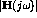.
7. What is the limit ofasgoes to zero?
8. What is the limit ofasgoes to infinity?
9. What is the corner frequency?
10. What isat the corner frequency?
11. Make a sketch showing the characteristics ofon a log-log plot. Label the slope of the curve where possible, the corner frequency, and the value ofat the corner frequency.
12. Describe the high and low frequency behavior in dB/octave.
1. Draw a passive LCR low-pass filter and write down the transfer function of your four-terminal network.
2. Determine approximations to the transfer function and filter corner frequency(s).
3. Write the resonance frequency,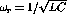, in terms of the corner frequency(s).
2. Write down the transfer function,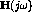, for the network shown below, and from it find:
1. the corner frequency(s) and
2. the value(s) ofat the corner frequency(s).
3. Sketchand the voltage phase-shift as a function of.
4. What type of filter is this?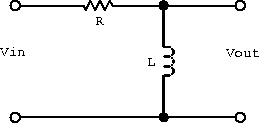1. What is the transfer function for the following circuit?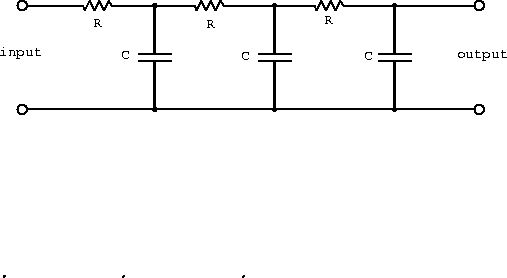2. Describe the frequency response at low and high frequencies?
3. Sketch the magnitudeon a log-log plot. (label slopes, the corner frequency(s), and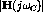)
4. What is the signal attenuation for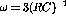.
1. Show that the transfer function of a single-pole RC filter drops by 3 db at the corner frequency. This is often refereed to as the 3 db down-point.
2. Design a bandpass RC filter with the transfer function shown below.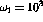rad/s and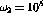rad/s are the 3 db down-points of the RC filter sections. Choose impedances so that the first section is not much affected by the loading of the second section.
3. Are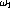and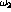the 3 db down-points of a bandpass filter?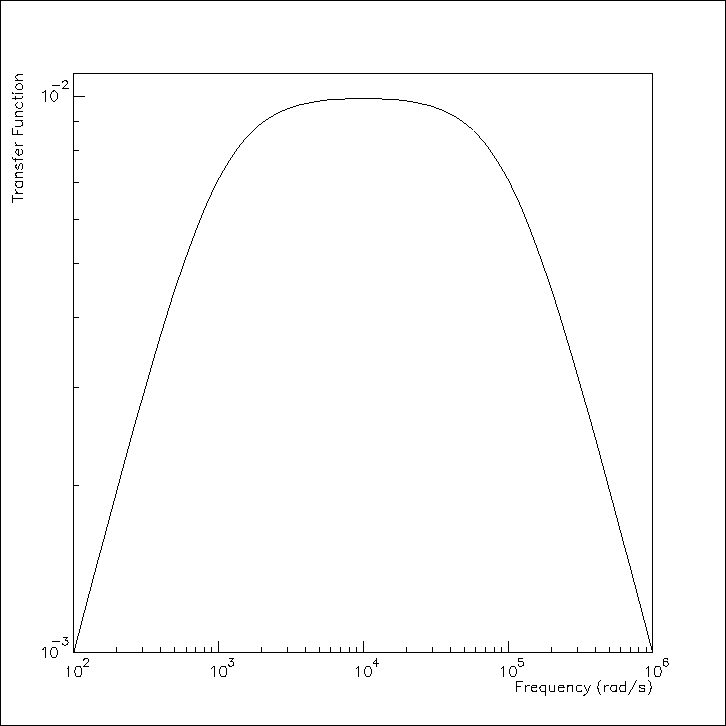Next: Diode Circuits Up: Filter Circuits Previous: Amplifier with Negative Feedback

Doug Gingrich
Tue Jul 13 16:55:15 EDT 1999